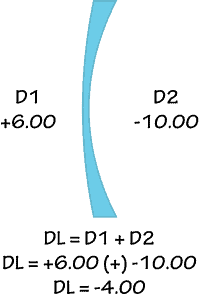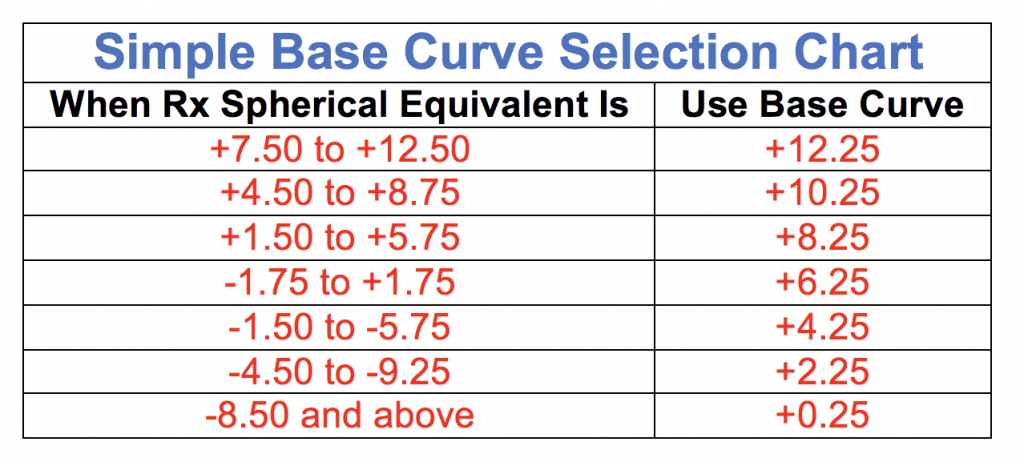Select Page

This lesson is under construction this week: 7/16/17 – 7/21/17

In optical theory it is said that the base curve is, “The curve from which all other curves are measured.”

In modern lens design we can think of the base curve as always being the front surface of the lens.

In modern lens design that curve is always plus (+) and has a convex shape.

The corresponding curves that create the actual lens power (bend light) are placed on the back of the lens, are minus (-) and have a concave shape.That relationship was covered in the previous lesson, The Nominal Lens Formula.

Matching a base curve to a prescription is part of surfacing. Surfacing is the actual grinding away of the plastic on a lens to create the curves necessary to match a prescription. These days surfacing is done at the “lab” not at the “store” and is no longer part of the day-to-day work of an optician.

Here is what I want you to know about base curve for your actual day-to-day work.

1) In an upcoming lesson you will learn about free-form lens design. Only a computer can determine the correct base curve on a lens designed using free-form technology. In fact most labs today will not allow you to change or request a base curve at all.

2) I want you to know that there are now lenses with variable base curve designs (the Camber series of progressive lenses from Younger). This completely overrides any use of the nominal lens formula or simple base curve calculations.

3) Never attempt to change the lens base curve to match a frame base curve. You may hear someone say, “Well, we will just run it on a ______ base instead.” Just smile and nod your head and then ignore them and have the job finished at a good lab with a high-end edger. A good edger can overcome many (but not all) poor frame and Rx matching problems. How to properly match lens and frame curves is covered in Course 3: All About Frames.

Here is what you will need to know about base curve theory for the ABO or any practical exams you might need to take.

Both the methods below work off the spherical equivalent of the given prescription. The formula to determine the spherical equivalent of any Rx is:

Rx sphere power added to 1/2 the cylinder power. If the Rx is a sphere like -2.50 then you work with the -2.50. Spherical equivalents are also used in other formulas and when working with contact lenses.

Don’t worry this will make more sense after you get to the very next lesson, Flat Transposition.

The most basic rule is that you always want the base curve to be as close to +6.00 as you can get and still have the Rx work. In theory +6.00 should always give you the best possible combination of curves for weight, optics, etc.

Study the chart below. You will want to remember that the higher the plus power, the higher plus the base curve will become. The more minus the power (less plus power to minus power) the lower plus the base curve will become. A high minus Rx may even have a base curve of +0.25 or even 0.00.

To do that you can use Vogel’s Rule which is this:

#### For a minus prescription: Base Curve = 1/2 the Rx spherical equivalent + 6.00D

Examples:

Choose the correct base curve for the following Rx: +4.50 -1.00 X 89
The spherical equivalent of that Rx is +4.00 [+4.50 (+) 1/2 of -1.00]
+4.00 (+) +6.00 = +10.00

Choose the correct base curve for the following Rx: -4.50 -1.00 X 89
The spherical equivalent of that Rx is -5.00 [-4.50 + 1/2 of -1.00]
1/2 of that spherical equivalent is -2.50 [-5.00/2]
-2.50 (+) +6.00 = +3.50

Another common method is to simply use a base curve chart. Just make sure you are reading it correctly. You will find charts have overlapping ranges so you must be careful and be sure you are choosing the curve that is closest to that +6.00.

A simple base curve chart might look like this:Examples:

Choose the correct base curve for the following Rx: +4.50 -1.00 X 89
The spherical equivalent of that Rx is +4.00 [+4.50 (+) 1/2 of -1.00]
+4.00 falls between +1.50 and +5.75 so our BC would be +8.25

Choose the correct base curve for the following Rx: -4.50 -1.00 X 89
The spherical equivalent of that Rx is -5.00 [-4.50 + 1/2 of -1.00]
-5.00 falls between -4.50 and -9.25
BUT it also falls between -1.50 and -5.75 and that BC is closer to our +6.00 so we choose +4.25 NOT +2.25.